# Parsing algorithm for Graph implementation part I

Working on your own project has the inconvenient that you have to find some extra time to do all this stuff. My last project (VLO Framework) it's been like a dream come true (Developing a graphic system is not easy). Maybe in two or three months I'll be able to sit back and reflect a bit, but for now it's pretty hectic. I'm keen on releasing a new product (P-Zaggy) that implements a lot of algorithms to use with graphs. I am preparing a power point that I'll publish in order to introduce you to this application. I have a lot of ideas, and sometimes it's quite difficult to tell my brain to shut up!.

In this article, I'll introduce you How I built this little parsing algorithm to implement a graph inside the canvas test framework. With this little gadget, now the application is getting quite spectacular. Now, adding a simple edges list, we can generate a diagram and using the layout algorithm, format the entire graph as we desire.

The system is very simple. We only need to create the adjacent list by adding the name of the couple of vertex. The more interesting thing is, that we can interact with the existing graph. We can create a piece of the graph, and then adding other section only referencing the name of the vertex.

I've been tweaking the layout properties with new parameters:
1. Show energy calculations (this parameter let you visualize the amount of kinetic energy calculated from the system in the output window)
2. Centre graph after finishing (this parameter will centre the graph in the middle of the canvas)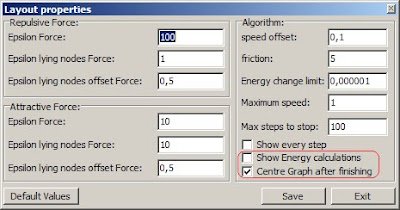Now going to Graph -> Graph Implementation, will display this form: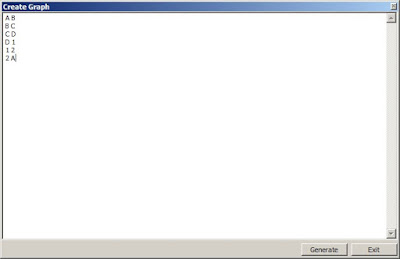After creating a simple edge list by using the following structure Vertex1 + " " + Vertex2. That means that vertex1 is connected to vertex2. If we generate the adjacent list from the image we'll get a randomly positioned graph: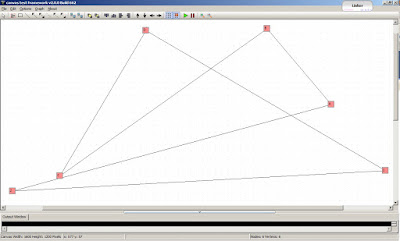If we use the layout algorithm to organize the graph, we'll get this:And we can keep playing with the parsing window, creating another graph and connecting this to the existing one: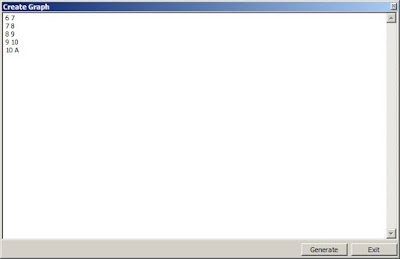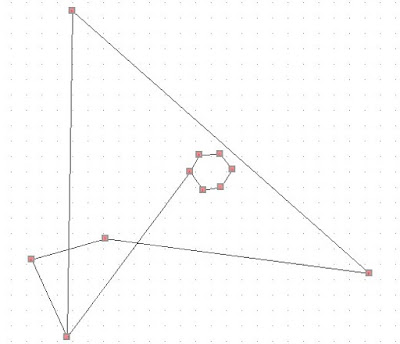Playing with the layout algorithm, the application will show the following picture: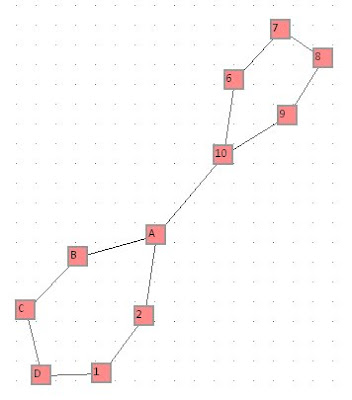Here there is a video showing the parsing window:

To the centre of the Graph, I've used the next algorithm:

`procedure TGraph.Centre;varCenter, CenterGraph: TPoint;Vertex1, Vertex2: TPoint;i: Integer;begin// Center of the CanvasCenter := point(FCanvas.width div 2, FCanvas.height div 2);Vertex1 := point(9999, 9999);Vertex2 := point(0, 0);for i := 0 to Boxlist.Count - 1 dobegin  if Boxlist.items[i].Vertex1.X < Vertex1.X then      Vertex1.X := Boxlist.items[i].Vertex1.X;  if Boxlist.items[i].Vertex1.Y < Vertex1.Y then      Vertex1.Y := Boxlist.items[i].Vertex1.Y;  if Boxlist.items[i].Vertex2.X > Vertex2.X then      Vertex2.X := Boxlist.items[i].Vertex2.X;  if Boxlist.items[i].Vertex2.Y > Vertex2.Y then      Vertex2.Y := Boxlist.items[i].Vertex2.Y;end;CenterGraph := point(((Vertex1.X + Vertex2.X) div 2), ((Vertex1.Y + Vertex2.Y) div 2));for i := 0 to Boxlist.Count - 1 do  Boxlist.items[i].Move(Center.X - CenterGraph.X, Center.Y - CenterGraph.Y);end;`

It consist in reading all the vertex, and move all the graph from its own centre to the centre of the canvas.

For the parsing window, the code looks like this:

`for i := 0 to Memo1.Lines.count - 1 dobegin    parsingList := TParsingList.Create;    parsingList.get(Memo1.Lines[i]);    if parsingList.count = 2 then    begin        id1 := parsingList.id1;        id2 := parsingList.id2;        box1 := boxManager.Boxlist.GetBoxByDescription(id1);        if not Assigned(box1) then        begin            box1 := Tbox.Create(id1, Vertex1, Vertex2);            boxManager.Boxlist.Add(box1);            boxManager.Boxlist.Sort(@Compare);        end;        box2 := boxManager.Boxlist.GetBoxByDescription(id2);        if not Assigned(box2) then        begin            box2 := Tbox.Create(id2, Vertex1, Vertex2);            boxManager.Boxlist.Add(box2);            boxManager.Boxlist.Sort(@Compare);        end;        if not boxManager.ConnectorList.existConnection(box1, box2) then        begin            concept := boxManager.getLineFactory(SimpleLine);            Conn := TConnector.Create(box1, box2, concept);            boxManager.ConnectorList.Add(Conn);            box1.AddNeighBour(box2.id);            box2.AddNeighBour(box1.id);        end;    end;    FreeAndNil(parsingList);end;`

Here I've left the last version of the compiled application:
If you spot anything on the program, don't hesitate to contact me, as I'm keen to answer any question. I hope you enjoy!.